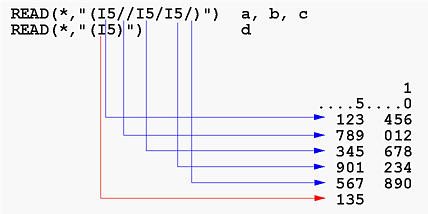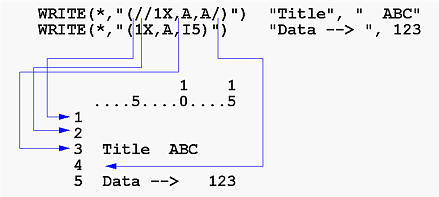# Vertical Spacing Control: /The slash edit descriptor / is used to terminate the current input/output line. The general forms of this descriptor are as follows:

/     and      r/

• For Input: The current input line is skipped and the remaining unread content on the current input line is ignored. The reading process starts at the first position on the next input line.
• For Output: The current output line is printed and the next output item, including the printer control character, starts at the first position of a new buffer output line.
• While commas are used for separating two neighboring edit descriptors, they are not required if one of these two neighboring edit descriptors is a /. For example, the following
```READ(*,"(I3,5X,I5,/,/,T15,F10.0)")  ......
```
is equivalent to
```READ(*,"(I3,5X,I5//T15,F10.0)")  ......
```
• r is equivalent to writing / r times. For example, 4/ is equivalent to ////.### Examples

• Consider the following input:
```         1
....5....0
123  456
789  012
345  678
```
```INTEGER :: a, b, c

```
The reading starts with the first input line. I5 is for reading an integer for a. Thus, a receives a value of 123. Since the next edit descriptor is a /, the content on the current input line is ignored. As a result, the next available position for b is the first position on the second input line and b receives 789. After this, we see a second / which causes ignoring the remaining of the second input line. Therefore, the value for c is taken from the first five positions on the third input line and c receives 345.

Note that the above READ statement consumes three input lines. The first line is read using the edit descriptors between the opening left parenthesis ( and the first /, the second input line is read using the edit descriptors between the two /s, and the third input line is read using the edit descriptors between the second / and the closing right parenthesis ).

• Consider the following input:
```         1
....5....0
123  456
789  012
345  678
901  234
567  890
135
```
```INTEGER :: a, b, c, d

```
a is read in using I5 from the first five positions of the first line. Thus, it receives 123. After this, we see a /, which terminates the use of the first input line and jumps to the second. Then, we see another /, which terminates the use of the second input line and jumps to the third. Now, we have I5 for variable b. Therefore, b receives 345 from the third input line. After reading b, we have a / and c will use the fourth input line. Hence, c receives 901. But, immediately after this I5, there is another / and as a result this fourth line is also skipped. Thus, we are on the fifth line. Then, we reach the end of the format and there is no variables remains. Thus, the first READ completes. Note that after the first READ completes, five lives have been read.

The above discussion is illustrated with the figure below.• Consider the following WRITE statement.
```WRITE(*,"(I5//I6/1X,A)")  123, 456, "+-*/"
```
When this WRITE starts, the format begins with I5. Since this is an edit descriptor that requires a value, the format scanning stops here and puts the value 123 into positions 1 to 5 of the first output line:
```         1
....5....0
123
```
After printing the value of 123, format scanning starts with /. This causes the end of the current line. Then, we see another /, which terminates the second output line. The next edit descriptor is I6. Since it is an edit descriptor that requires a value, 456 is printed in positions 1 to 6 on the third output line:
```         1
....5....0
123

456
```
Then, format scanning resumes with /, which terminates the third output line. The next is 1X, followed by A. Hence, the first position of the next (i.e., fourth) output line is a space (i.e., 1X), followed by +-*/. After printing this item, the end of format is reached. Since all data items have been printed, this WRITE completes. In summary, this WRITE prints four output lines. Of these four lines, the second one is a blank line generated by the two adjacent slashes.
```         1
....5....0
123

456
+-*/
```
• Because the use of / can print many lines with a single WRITE and a single format, this edit descriptor is frequently used for combining the table title and heading together into a single format. The following is a simple example:
```WRITE(*,"(//1X,A,A/)")  "Title", "  ABC"
WRITE(*,"(1X,A,I5)")    "Data --> ", 123
```
When the first WRITE starts, format scan begins with the first /. This terminates the first output line. Then, we see a second / which terminates the second line. Thus, we have two blank lines. The next edit descriptor is 1X, which moves to position 2 of the third output line. Now, we see A, which requires a data value. Therefore, "Title" is printed in positions 2 to 6 on the third line:
```         1
....5....0
<--- line 1, printed by /
<--- line 2, printed by /
Title
```
Format scanning continues and encounters the second A. Thus, "  ABC" is printed in positions 7 to 11. Then, we see a / which terminates the third output line. After this, the end of format is reached and no data items remains, the first WRITE completes with the fourth output line being a blank one:
```         1    1
....5....0....5
<--- line 1, printed by /
<--- line 2, printed by /
Title  ABC     <--- line 3, printed by 1X, A, A/
<--- line 4, printed by the closing parenthesis
```
The second WRITE prints its content on the fifth line and the final result is shown below:
```         1    1
....5....0....5

Title  ABC

Data -->   123 <--- line 5, printed by 1X, A, I5
```
In summary, the first WRITE prints four lines. Of these four, the first is generated by the opening left parenthesis and the first slash, the second is generated by the two adjacent slashes, the third is generated by 1X,A,A/, and the fourth is generated by the third slash and the closing parenthesis. Note that the first, second and fourth output lines are blank lines.

The above discussion is summarized in the figure below: